# Torque

## Introduction

Torque will be used to quantify forces applied to bodies which can rotate.

Previously, in the particle model, it didn’t matter where on a body we applied a force. However, with rigid bodies, that is no longer true.

(Things are no longer point particles.)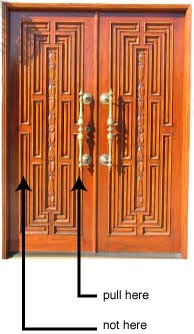## Torque

1. The magnitude of the force
2. The distance r, from the pivot point to where the force is applied
3. The angle at which the force is applied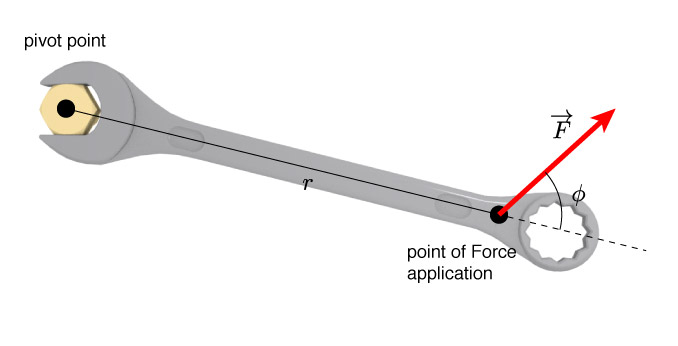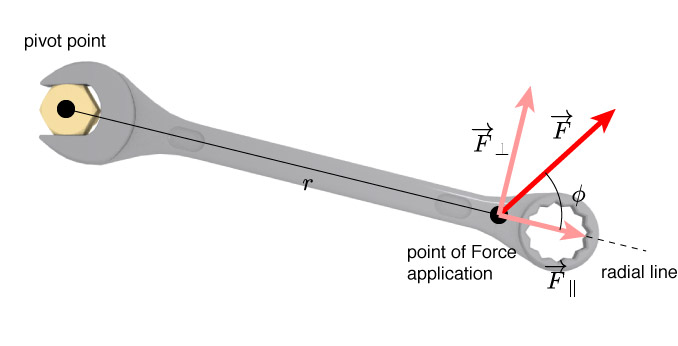$F_\perp$ contributes to rotating the wrench

$F_\parallel$ does not contribute to the rotation at all.

We know from experience that

1. it’s easier to open a door when you push at the point that’s farthest from the hinges
2. you have to push in the direction you want it to go.

(let’s turn that into math)Torque, $\tau$ will be given by:

$$\tau = r F_\perp$$Find $F_\perp$ based on $\overrightarrow{F}$ and $\phi$### Lever arm changes

As the angle of the line of action changes, then the length of the 'lever arm' changes.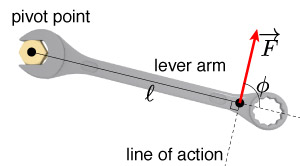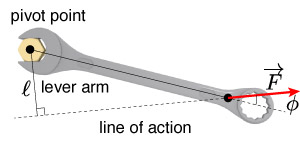$$\tau = F \mathcal{\ell}$$

#### In general...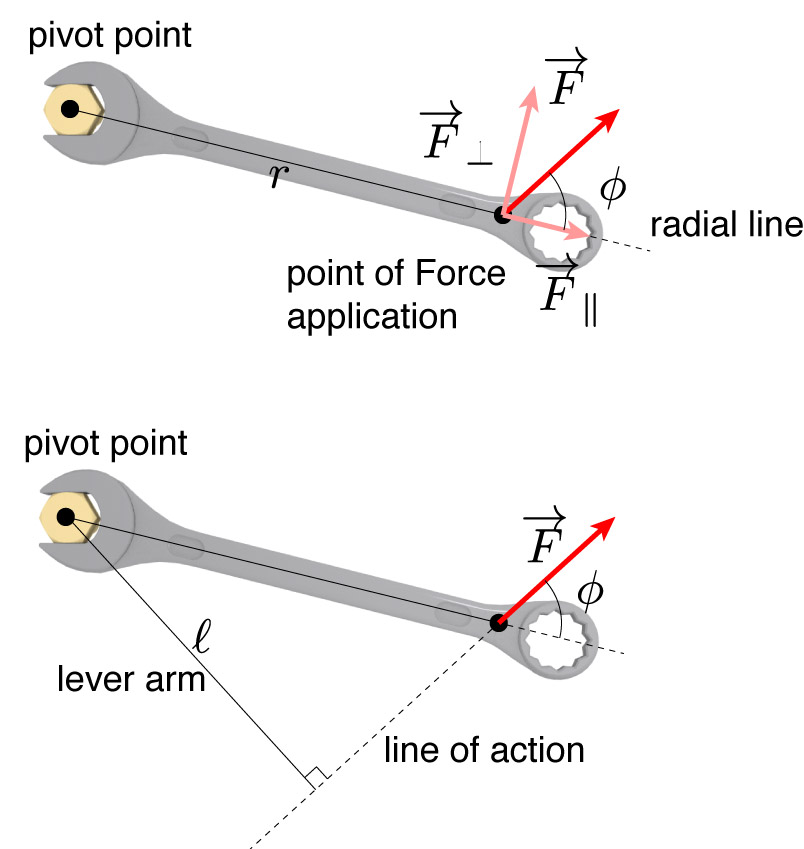Either way you set it up, the torque is still given by the force applied, times the distance away from the pivot point, and the sine of the angle between the force and the radius of motion.

$$\tau = r F_\perp = r F \sin \phi$$

or,

$$\tau = F \mathcal{\ell} = F r \sin \phi$$

Which force will provide the largest magnitude of torque on the wrench? (E if torque is the same for each.)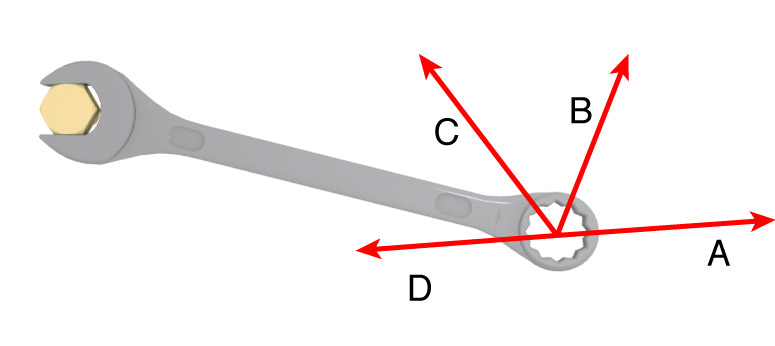## Net Torques

Just like the net force, we can add up all the different torques applied to an object to see if, and which way, it will rotate.$$\sum \tau = \tau_1 + \tau_2 + \tau_3 \ldots$$What is the net torque around the labeled axis?

#### Torque

Torque, like force, is a vector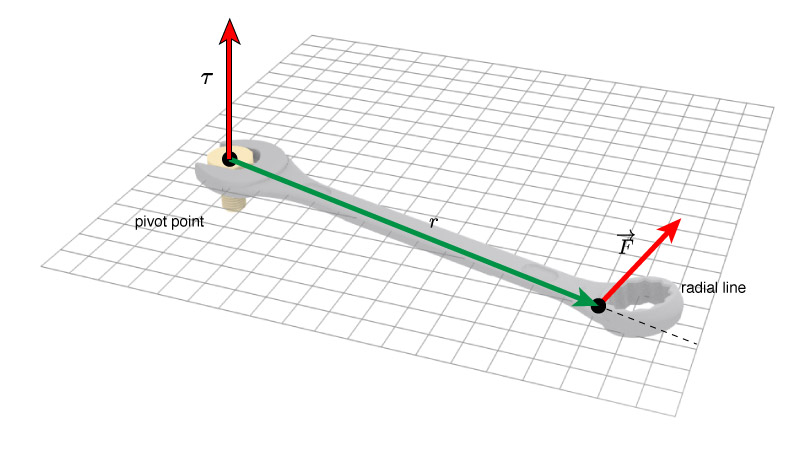$$\bbox[10px ,border:3px solid red]{\tau = |r||F|\sin\phi}$$ $$\bbox[10px ,border:3px solid red]{\tau = \mathbf{r} \times \mathbf{F}}$$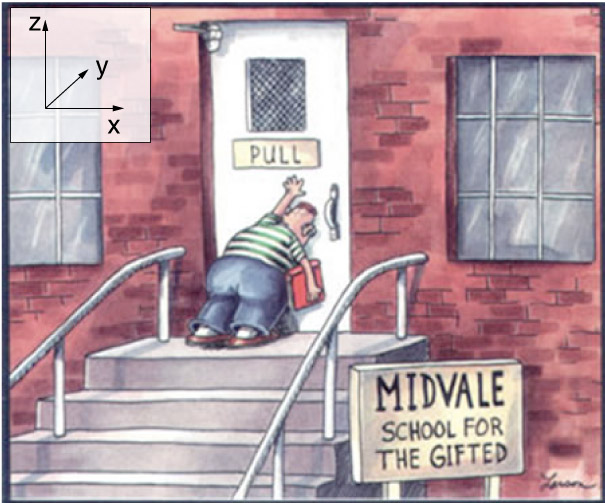To open the door, which direction should the torque vector point?

1. $\mathbf{\hat{i}}$
2. $-\mathbf{\hat{i}}$
3. $\mathbf{\hat{j}}$
4. $-\mathbf{\hat{j}}$
5. $\mathbf{\hat{k}}$
6. $-\mathbf{\hat{k}}$To open the door, which direction should the torque vector point?

1. $\mathbf{x}$
2. $-\mathbf{x}$
3. $\mathbf{y}$
4. $-\mathbf{y}$
5. $\mathbf{z}$
6. $-\mathbf{z}$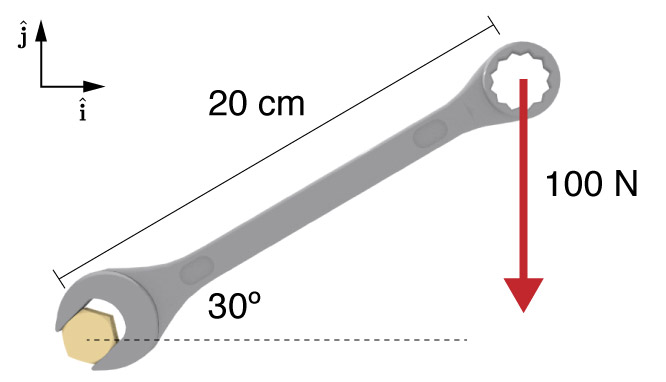What is the torque applied to the nut?What is the torque applied to the nut? (use unit vectors)

#### Fulcrums and Levers

Let's balance a board on a fulcrum.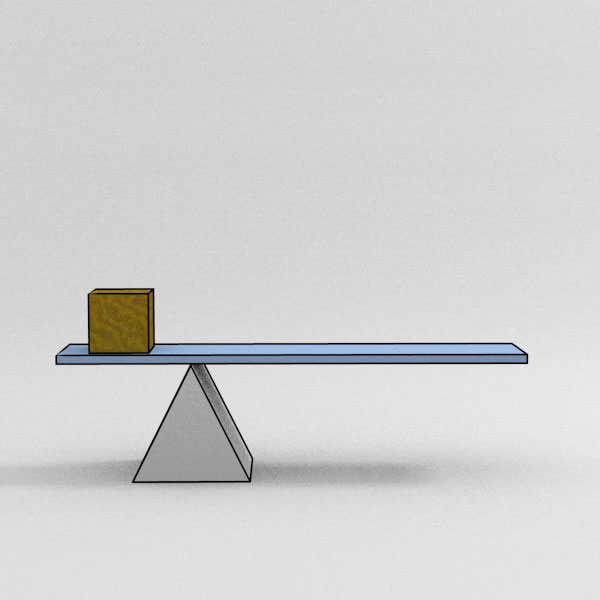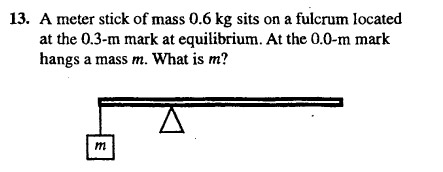A meter stick of mass 0.6 kg sits on a fulcrum located at the 30 cm mark at equilibrium. At the end of the stick (0.0 cm) hangs a mass m. What is m?

1. A force of some N is applied...? (3 parts)

2. What is the cross product of these two vectors...? (3 parts)

Pulley B hangs from the ceiling and has a diameter d. A string twined about the pulley leads around pulley A, hanging from the ceiling, and to a mass M. A beam of length L is attached to the pulley B itself and stretches out horizon- tally. A mass m is connected to the end. The system is in static equilibrium. (See figure.)## Moment of Rotational Inertia

We saw before that a force caused a linear acceleration, with Newton’s 2 ndlaw:

$$F = ma$$

Since a torque is basically the rotational equivalent of force, then it should cause an angular acceleration.

$$\tau \rightarrow \alpha$$

However, we need a constant of proportionality between the two terms.$$\bbox[10px, border:2px solid red]{\tau = I \alpha}$$

$I$ is called the 'moment of inertia'.

It will describe how easy or hard it is to rotate a rigid body. (just like mass told us how hard or easy it was to move a body)

In the case of F, and linear motion, if we applied the same force to two different masses, then the heavier mass would have a smaller acceleration.

But, in the case of rotating objects, it’s not just the mass that affects the angular rotation, but also how that mass is distributed.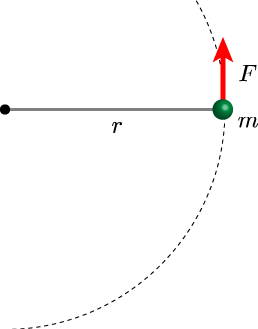In this case, the 2nd law for this particle with mass $m$, held a distance $r$ from the center will be: $$a_T = \frac{F}{m}$$

but the tangential acceleration is related to the angular acceleration by $a_T = \alpha r$. So we can write: $$\alpha = \frac{F}{mr}$$

Using also $\tau = r F_\perp$, we can write this as: $$\alpha = \frac{\tau}{mr^2}$$Thus we have for a ball rotating around an axis: $$\alpha = \frac{\tau}{mr^2}$$

For this geometry, the moment of inertia, $I$, is given by: $$I = mr^2$$

This makes sense, if the mass is heavier, then the angular acceleration will be less, and also if mass is farther away from the center, the angular acceleration will be less.We can simply sum up all the moments of inertia for each little particle:

$$I = m_1 r_1^2 + m_2 r_2^2 + m_3 r_3^2 \ldots = \sum mr^2$$

#### Example

Calculate the moment of inertias for this shape based on the two axes shown.

(m1 = 1.0 kg, m2 = 1.5 kg and m3 = 1.0 kg)For shapes of arbitrary dimensions, things would get a little more complicated.

Even for a seemingly simple shape like a thin rod being rotated around one of its ends, we'd have to do some integral calculus to figure out the moment of inertia:

For discrete masses: $$I = \sum m_i r_i^2$$

For continuous masses: $$I = \int r^2 dm$$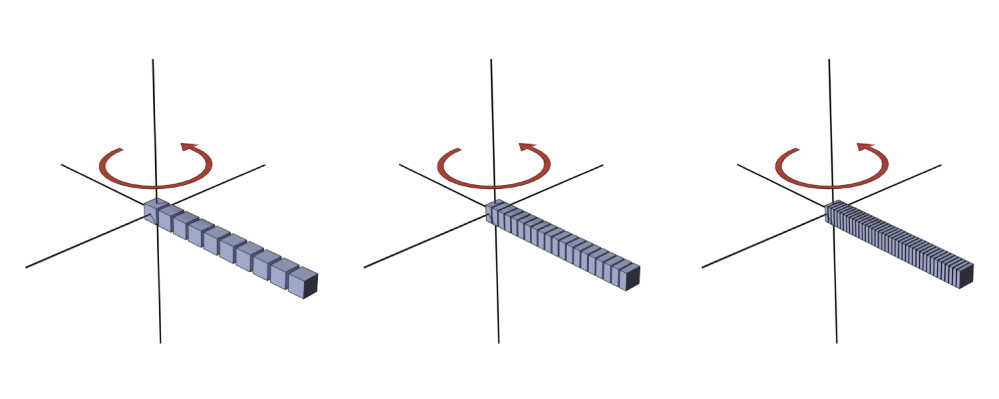### Moment of Inertia for Arbitrary Shapes

We'll begin by integrating the mass of the object:

$$I = \int r^2 dm$$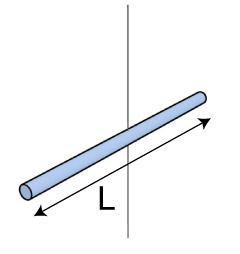Here is a uniform rod of total mass $M$. Express the mass element $dm$ in terms of $dx$:

1. $$dm = \frac{L}{M}dx$$
2. $$dm = \frac{M}{L}dx$$
3. $$dm = M L \; dx$$
4. $$dm = \frac{L M}{dx}$$

#### Rotate a rod:To calculate $I$ for this geometry:

$$I = \int r^2 dm$$

We'll need to express $dm$ in terms of $x$ (position.)

$$dm = \frac{M}{L} dx$$

We can integrate over dx

$$\int_{x = -L/2}^{x=+L/2} x^2 \frac{M}{L}dx$$

After integrating and evaluating:

$$I = \frac{1}{12}ML^2$$

### Parallel Axis Theorem

This will be easy for simple shapes, but in the case of more complex shapes, or less obvious axes of rotation, we might need the parallel axis theorem.

$$I = I_\textrm{com} + M h^2$$

Here, $I_\textrm{com}$ is the moment of inertia through the center of mass, and $h$ is the distance between that axis and our axis of interest.

#### Rotate a rod (around and end)In the case of a rotation axis located at one of the ends of the rod, we can use the parallel axis theorem. (The new axis at the end is parallel to the com axis.

$$I = I_\textrm{com} + M h^2$$

We know the $I$ though the center of mass:

$$I = \frac{1}{12}ML^2 + Mh^2$$

And the distance to the end is $L/2$:

$$I = \frac{1}{12}ML^2 + M\left(\frac{L}{2}\right)^2 = \frac{1}{3}ML^2$$

#### Moment of inertia for various geometries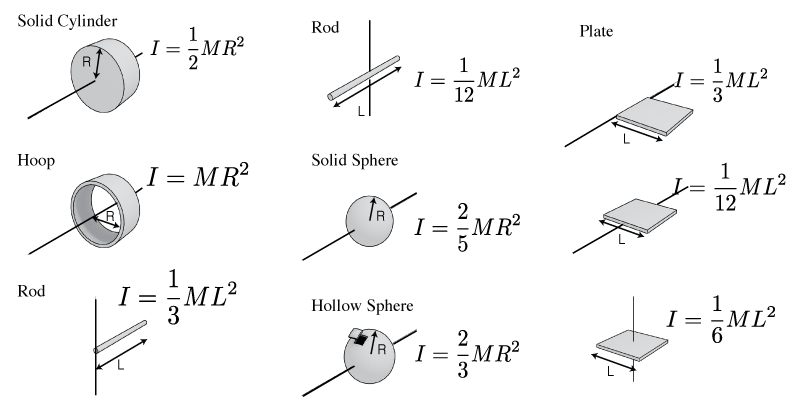The engine in a plane can deliver 500 N$\cdot$m to the propeller. The propeller has a mass of 40 kg and is 2.0 meters long (diameter). How long does it take to reach 2000 rpm?

A telephone pole falls over in a storm. It is 7.0 meters tall and has a mass of 260 kg. Estimate the angular acceleration of the pole when it has fallen by $25^\circ$ from the vertical.

a) What would the moment of inertia be for a regular wooden door...?

b) ...what will its angular acceleration be?

#### Energy of Rotation

The total mechanical energy of a system was the kinetic plus potential

$$E_\textrm{mech} = KE + U$$

However, $KE$ needs to include both the translational energy: $\frac{1}{2}mv^2$ and the rotational energy:

$$KE_\textrm{rot} = \frac{1}{2}I \omega^2$$

thus

$$E_\textrm{mech} = \frac{1}{2}m v^2 + \frac{1}{2}I \omega^2 + mgh$$

Which object wins the race?

1. The Solid Cylinder
2. The Empty Hoop
3. It'll be a tie

Which can wins the race?

1. Chicken Broth
2. Cream 'O Chicken
3. It'll be a tie

## Tale of Two Energies

This combination of motion demands that we account for both linear and rotational energy when describing a rolling object:

$$K_\textrm{rolling w.o. slipping} = \frac{1}{2}I\omega^2 + \frac{1}{2} M v^2$$

Just to be more specific

$$K_\textrm{rolling w.o. slipping} = \frac{1}{2}I_\textrm{com}\omega^2 + \frac{1}{2} M v_\textrm{com}^2$$

We'll often need the geometrical constraint: $v = \omega r$ to deal with these two terms.

## Rolling and friction

We can make a free body diagram of a rolling ball.The forces acting on the ball:

1. Weight (gravity)
2. Normal force (perp to ramp)
3. static friction (points up the ramp)Which force creates a non-zero torque?

1. Static Friction
2. Gravity
3. Normal Force
4. They all create torques

#### What's the torque?Starting with the rotational version of Newton's law:

$$\tau = I \alpha$$

we can consider the forces that will contribute to a rotation of the disk.The only force acting with a perpendicular component is friction.

$$Rf_s = \tau = I \alpha$$

Since $\alpha = -a_\textrm{com}/R$

$$f_s = -I_\textrm{com} \frac{a_\textrm{com}}{R^2}$$But, we can also consider the sum of forces along the surface of the ramp:

$$\Sigma F_\parallel = {F_G}_\parallel + \textrm{friction} = m a_\textrm{com}$$

Plug some stuff in:

$$Mg\sin \theta - I_\textrm{com}\frac{a_\textrm{com}}{R^2} = ma_\textrm{com}$$

Rearrange for $a_\textrm{com}$:

$$a_\textrm{com} = \frac{g \sin \theta}{1+ I_\textrm{com}/MR^2}$$

A spherical shell rolls down a ramp starting at height $h$. What is the speed of the sphere at the bottom?

#### Bucket

A bucket is attached to a string that is wrapped around a cylinder as shown. If the bucket is released from rest 1 meter above the ground, how long will it take to hit the floor?
(Mass of the bucket = 2.0 kg, mass of cylinder = 1 kg, radius of cylinder = 2.0 cm, mass of string = 0 kg)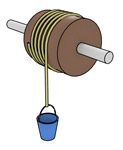## Conservation of Angular Momentum

Magnitude $$L = I \omega$$

Direction $$\mathbf{L} = I \overrightarrow{\omega}$$

Conservation: $$L_i = L_f$$

A kid (mass = 36 kg) stands at the center of a rotating disk (a.k.a merry-go-round). Its mass is 200 kg and rotates once every 2.5 seconds. If the kid walks 2.0 meters away from the center to reach the edge, what will the period of rotation be when he reaches the edge?

#### Angular Momentum if a Particle

If a particles has a linear momentum, the we might expect it to also have an angular momentum? $$\mathbf{L} = \mathbf{r} \times \mathbf{p}$$ This quantity is conserved.

Here, $\mathbf{r}$ is the distance away from the 'origin' and $\mathbf{p}$ is the momentum vector.

### Angular Momentum - Particle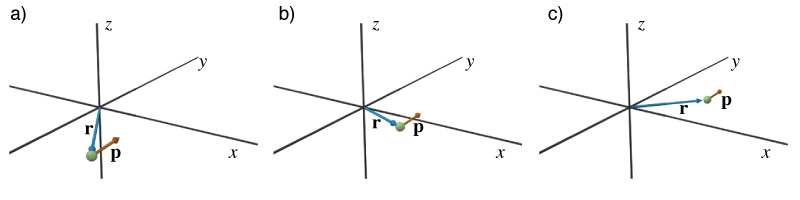#### Angular momentum of a satellite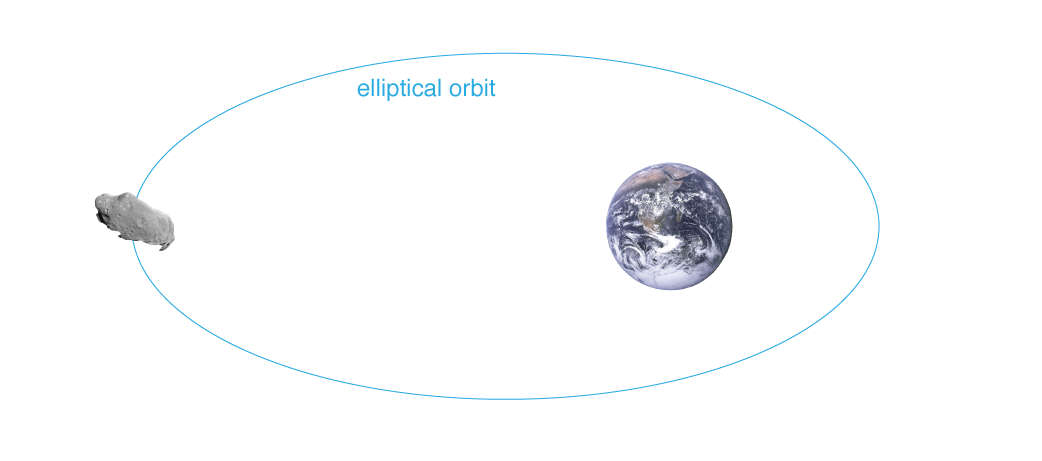#### Ballistic Pendulum

A 2.0 kg block hangs from the end of a 1.5 kg, 1.0 meter long rod, together forming a pendulum that swings from a frictionless pivot at the top end of the rod. A 10 g bullet is fired horizontally into the block, where it sticks, causing the pendulum to swing out to a 30° angle. What was the speed of the bullet? (Solve using ang. momentum)

#### The 2nd Law

Earlier, we rephrased $\mathbf{F} = m\mathbf{a}$ as $\mathbf{F} = \frac{d\mathbf{p}}{dt}$

We can consider the rotational equivalent to this law:

$$\overrightarrow{\tau}_\textrm{net} = \frac{d \mathbf{L}}{dt}$$

#### The 2nd Law

Earlier, we rephrased $\mathbf{F} = m\mathbf{a}$ as $\mathbf{F} = \frac{\Delta \mathbf{p}}{\Delta t}$

We can consider the rotational equivalent to this law:

$$\overrightarrow{\tau}_\textrm{net} = \frac{\Delta \mathbf{L}}{\Delta t}$$

## Gyroscope

We saw that the simple gyroscope in general stays upright.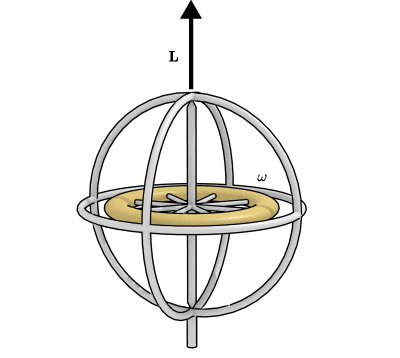Conservation of angular momentum says that in the absence of external torques, the angular momentum vector will not change.

#### A fallen Gyro

In the case of a non-spinning gyroscope, the torque due gravity will cause the gryo to fall over, without delay.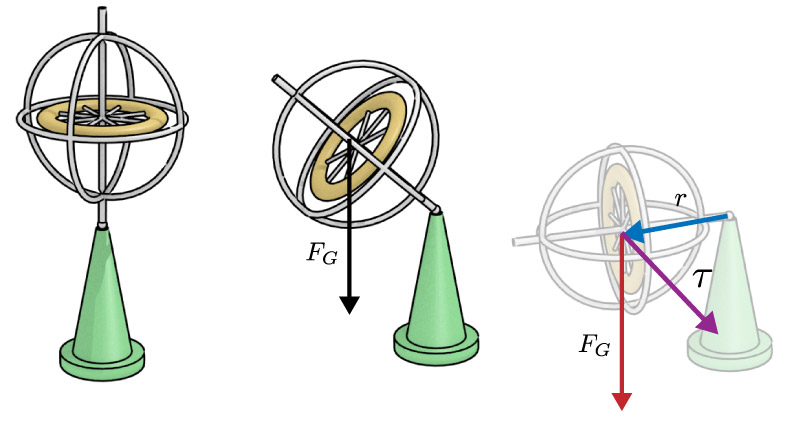#### A spinning gyro

If however, the gyroscope is spinning.Now, there is an external torque (due to gravity) so it will change the angular momentum. But, we can't use the torque to change the $\omega$ of the gyroscope. (it's $\perp$ to $\omega$) Thus, the magnitude of the $\mathbf{L}$ won't change, but the direction will.

#### PrecessionThis change in the direction of $\mathbf{L}$ (and $\omega$) is called the gryoscopic precession.The rate of precession can be calculated by considering the torque:

$$\overrightarrow{\tau} = \frac{d\overrightarrow{L}}{dt} \Rightarrow d\overrightarrow{L} = \overrightarrow{\tau}dt$$

But, the torque is just $mgr$$$L d \phi = d L = mgr dt$$

Thus, the angular precession rate $\Omega = d\phi/dt$ can be given by:

$$\Omega = \frac{mgr}{I \omega}$$